# JAC Class 9 Maths Solutions Chapter 8 Quadrilaterals Ex 8.1

Jharkhand Board JAC Class 9 Maths Solutions Chapter 8 Quadrilaterals Ex 8.1 Textbook Exercise Questions and Answers.

## JAC Board Class 9th Maths Solutions Chapter 8 Quadrilaterals Ex 8.1

Page-146

Question 1.
The angles of quadrilateral are in the ratio 3 : 5 : 9 : 13. Find all the angles of the quadrilateral.
Let the angles be 3x, 5x, 9x and 13x.
Sum of the interior angles of the quadrilateral = 360°
Now, 3x + 5x + 9x + 13x = 360°
⇒ 30x = 360°
⇒ x = 12°
3x = 3 × 12° = 36°
5x = 5 × 12° = 60°
9x = 9 × 12° = 108°
13x= 13 × 12°= 156°

Question 2.
If the diagonals of a parallelogram are equal, then show that it is a rectangle.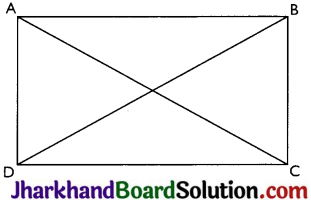Given: AC = BD
To prove: ABCD is a rectangle.
Proof: In order to prove ABCD is a rectangle we need to prove that one of its interior angle is right angle.
BA = BA (Common)
BC = AD (Opposite sides of a parallelogram are equal)
AC = BD (Given)
Therefore, ΔABC = ΔBAD by SSS congruence criterion.
⇒ ∠A = ∠B (by CPCT)
Also, ∠A + ∠B = 180° (Sum of the angles on the same side of the transversal)
⇒ 2∠A = 180°
⇒ ∠A = 90° = ∠B
Thus, ABCD is a rectangle.Question 3.
Show that if the diagonals of a quadrilateral bisect each other at right angles, then it is a rhombus.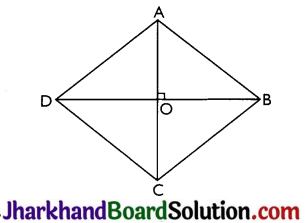Let ABCD be a quadrilateral whose diagonals bisect each other at right angles.
Given: OA = OC, OB = OD and ∠AOB = ∠BOC = ∠COD = ∠DOA = 90°
To prove: ABCD is parallelogram and AB = BC = CD = AD
Proof: In ΔAOB and ΔCOB,
OA = OC
∠AOB = ∠COB = 90°
OB = OB (Common)
Therefore, ΔAOB ≅ ΔBOC by SAS congruence criterion.
Thus, AB = BC (by CPCT)
Similarly, we can prove,
AB = BC = CD = AD Opposites sides of a quadrilateral are equal hence ABCD is a parallelogram.
Thus, ABCD is rhombus.

Question 4.
Show that the diagonals of a square are equal and bisect each other at right angles.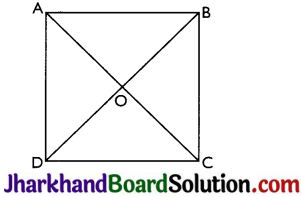Given: Let ABCD be a square and its diagonals AC and BD intersect each other at O.
To prove: AC = BD, AO = OC and ∠AOB = 90°
AB = BA (Common)
∠ABC = ∠BAD = 90° (Each angle of square is of 90°)
BC = AD (All sides of square are equal)
Therefore, ΔABC ≅ ΔBAD by SAS congruence criterion.
Thus, AC = BD (by CPCT).
i.e., diagonals are equal.

Now, In ΔAOB and ΔCOD,
∠BAO = ∠DCO (Alternate interior angles)
∠AOB = ∠COD (Vertically opposite angles)
AB = CD (Given)
Therefore, ΔAOB = ΔCOD by AAS congruence criterion.
Thus, AO = CO and BO = DO by CPCT i.e.
Diagonals bisect each other.
Now, In ΔAOB and ΔCOB,
OB = OB (common)
AO = CO (Proved above)
AB = CB (Sides of the square)
Therefore, ΔAOB ≅ ΔCOB by SSS congruence criterion.
∴ ∠AOB = ∠COB (CPCT)
Also, ∠AOB + ∠COB = 180°
(Linear pair)
Thus, ∠AOB = ∠COB = 90° i.e.
Diagonals bisect each other at right angles.

Question 5.
Show that if the diagonals of a which diagonals AC and BD bisect each other at right angles at O.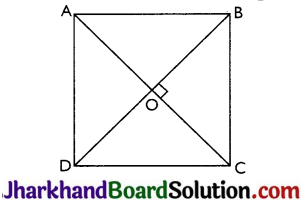Given: Let ABCD be a quadrilateral in which diagonals AC and BD bisect each other at right angles at O.
To prove: Quadrilateral ABCD is a square.
Proof: In ΔAOB and ΔCOD,
AO = CO (Diagonals bisect each other)
∠AOB = ∠COD (Vertically opposite angles)
OB = OD (Diagonals bisect each other)
Therefore, ΔAOB ≅ ΔCOD by SAS congruence criterion.
Thus, AB = CD by CPCT. ……. (i)
Now, In ΔAOD and ΔCOD,
AO = CO (Diagonals bisect each other)
∠AOD = ∠COD = 90° (Diagonals bisect each other at right angle)
OD = OD (Common)
Therefore, ΔAOD ≅ ΔCOD by SAS congruence criterion.
Thus, AD = CD by CPCT
Similarly, ΔCOD ≅ ΔBOC and BC = CD (CPCT)
⇒ AD = BC = CD = AB ……… (ii)
Also, as diagonals bisect each other, so, ABCD is a parallelogram.

Consider ΔACD and ΔBDC
CD = CD (Common)
AC = BD (Given)
⇒ ΔACD ≅ ΔBDC (by SSS congruence criterion)
As ABCD is a parallelogram so,
⇒ ∠ADC + ∠BDC = 180° (Angles on the same side of transversal)
∴ ∠ADC – ∠BCD = 90°.
So, ABCD is a parallelogram in which all sides are equal and one angle is 90°
⇒ ABCD is a square.Question 6.
Diagonal AC of a parallelogram ABCD bisects ∠A (see Fig.). Show that
(i) it bisects ∠C also,
(ii) ABCD is a rhombus.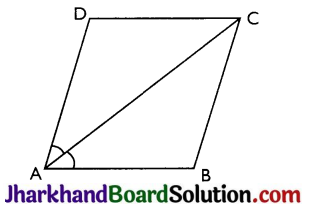(i) As ABCD is a parallelogram
⇒ AB || CD and AD || BC
⇒ ∠BAC = ∠ACD …….(i)
(Alternate interior angles)
(Alternate interior angles)
Also, ∠CAD = ABAC …….. (iii)
(As AC bisects ∠A)
Therefore, ∠BCA=∠DCA [By (i), (ii), (iii)]
⇒ AC bisects ∠C.

(ii) As ABCD is a parallelogram
⇒ AD = BC and AB = CD …(iv)
Also, as ∠CAD = ∠ACB (Alternate interior angles)
and ∠ACB = ∠ACD (Proved)
(sides opposites to equal angles are equal)
Similarly, AB = BC …(vi)
Therefore, AB = BC = CD = AD (By (iv), (v) (vi))
So, ABCD is a parallelogram in which all sides are equal.
⇒ ABCD is a rhombus.

Question 7.
ABCD is a rhombus. Show that diagonal AC bisects ∠A as well as ∠C and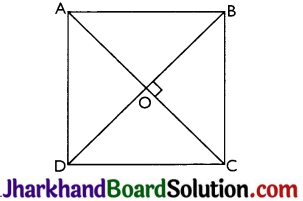Let ABCD is a rhombus and AC and BD be its diagonals.
Proof: In ΔACD – AD = CD (Sides of a rhombus)
∠DAC = ∠DCA
(Angles opposite to equal sides of a triangle are equal)
Also, AB || CD (Opposite sides of rhombus are parallel)
⇒ ∠DAC = ∠BCA (Alternate interior angles)
⇒ ∠DCA = ∠BCA
Therefore, AC bisects ∠C.
In ∆ABC,
AB = BC (Sides of a rhombus)
⇒ ∠BAC = ∠BCA (Angles opposite to equal sides are equal)
Also, AD || BC (Opposite sides of rhombus are parallel)
⇒ ∠DAC = ∠BCA (Alternate interior angles)
∴ ∠BAC = ∠DAC
⇒ AC bisects ∠A
Similarly we can prove that diagonal BD bisects ∠B as well as ∠D.

Question 8.
ABCD is a rectangle in which diagonal AC bisects ∠A as well as ∠C. Show that:
(i) ABCD is a square
(ii) diagonal BD bisects ∠B as well as ∠D.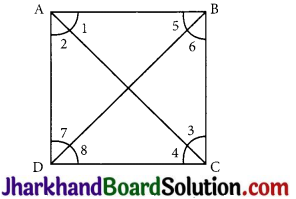(i) AB || CD and AD || BC (∵ opposite sides of rectangle are parallel)
⇒ ∠1 = ∠4 and∠2=∠3 (Alternate interior angles)
Also, as AC bisects ∠A and ∠C
⇒ ∠1 = ∠2 and ∠3 = ∠4
Therefore ∠2 = ∠4
So, in ΔACD,
AD = CD (sides opposite to equal angles are equal)
Also, AD = BC and AB = CD (∵ opposite sides of rectangle are equal)
∴ AB = BC = CD = AD
⇒ ABCD is a rectangle in which all sides are equal
⇒ ABCD is a square.

(ii) In ΔABD,
AB = AD (sides of a square)
⇒ ∠5 = ∠7 (Angles opposite to equal sides are equal)
Also, as AB || CD
⇒ ∠5 = ∠8 (Alternate interior angles)
∴ ∠7 = ∠8
⇒ BD bisects ∠D
Similarly, BD bisects ∠B.

Page-147

Question 9.
In parallelogram ABCD, two points P and Q are taken on diagonal BD such that DP = BQ (see Fig). Show that: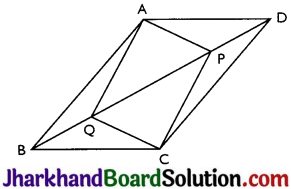(i) ΔAPD ≅ ΔCQB
(ii) AP = CQ
(iii) ΔAQB ≅ ΔCPD
(iv) AQ = CP
(v) APCQ is a parallelogram
(i) In ΔAPD and ΔCQB,
DP = BQ (Given)
∠ADP = ∠CBQ (Alternate interior angles)
AD = BC (Opposite sides of a ||gm)
Thus, ΔAPD ≅ ΔCQB
(by SAS congruence criterion)

(ii) AP = CQ by CPCT as ΔAPD ≅ ΔCQB.

(iii) In ΔAQB and ΔCPD,
BQ = DP (Given)
∠ABQ = ∠CDP (Alternate interior angles)
AB = CD (Opposite sides of a ||gm)
Thus, ΔAQB ≅ ΔCPD by SAS congruence criterion.

(iv) AQ = CP by CPCT as ΔAQB ≅ ΔCPD.

(v) From (ii) and (iv), we get opposite sides of quadrilateral APCQ are equal. Thus, APCQ is a ||gm.Question 10.
ABCD is a parallelogram and AP and CQ are perpendiculars from vertices A and C on diagonal BD (see Fig). Show that
(i) ΔAPB ≅ ΔCQD
(ii) AP = CQ(i) In ΔAPB and ΔCQD,
∠ABP = ∠CDQ (Alternate interior angles)
∠APB = ∠CQD = 90° (∵ AP and CQ are perpendiculars)
AB = CD (Opposite sides of a is a parallelogram)
Thus, ΔAPB ≅ ΔCQD (by AAS congruence criterion)

(ii) AP = CQ by CPCT as ΔAPB ≅ ΔCQD.

Question 11.
In ΔABC and ΔDEF, AB = DE, AB || DE, BC = EF and BC || EF. Vertices A, B and C are joined to vertices D, E and F respectively (see Fig).
Show that
(i) quadrilateral ABED is a parallelogram
(ii) quadrilateral BEFC is a parallelogram
(iv) quadrilateral ACFD is a parallelogram
(v) AC = DF
(vi) ΔABC ≅ ΔDEF.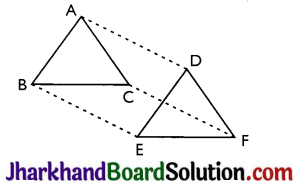(i) AB = DE and AB || DE (Given)
Thus, quadrilateral ABED is a parallelogram because a pair of opposite sides of a quadrilateral are equal and parallel to each other.

(ii) Again BC = EF and BC || EF.
Thus, quadrilateral BEFC is a parallelogram.

(iii) Since ABED and BEFC are parallelograms.
⇒ AD = BE and BE = CF
(Opposite sides of a parallelogram are equal)
Also, AD || BE and BE || CF
(Opposite sides of a parallelogram are parallel)

(iv) AD and CF are opposite sides of quadrilateral ACFD which are equal and parallel to each other.
Thus, ACFD is a parallelogram.

(v) AC || DF and AC = DF because ACFD is a parallelogram.

(vi) In ∆ABC and ∆DEF,
AB = DE (Given)
BC = EF (Given)
AC = DF (Opposite sides of a parallelogram ACFD)
Thus, ∆ABC ≅ ∆DEF (by SSS congruence criterion)Question 12.
ABCD is a trapezium in which AB || CD and AD = BC.
Show that
(i) ∠A = ∠B
(ii) ∠C = ∠D
(iv) diagonal AC = diagonal BD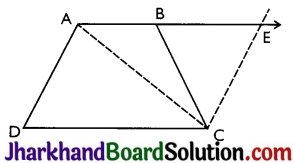[Hint: Extend AB and draw a line through C parallel to DA intersecting AB produced at E.]
Construction: Draw a line through C parallel to DA intersecting AB produced at E.
Proof:
AE || CD (as AB || CD)
∴ ∆DCE is a parallelogram as opposite sides are parallel
CE = AD (Opposite sides of a parallelogram)
Therefore, BC = CE
⇒ ∠CBE = ∠CEB
(Angles opposite to equal sides)
also, ∠A + ∠CBE = 180°
(Angles on the same side of transversal and ∠CBE = ∠CEB)
∠B + ∠CBE = 180° (Linear pair)
⇒ ∠A = ∠B

(ii) ∠A + ∠D = ∠B+ ∠C= 180° (Angles on the same side of transversal)
⇒ ∠A + ∠D = ∠A + ∠C (∵ ∠A = ∠B)
⇒ ∠D = ∠C